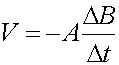Formulas for Induction

To discuss induction, it is first necessary to define the magnetic flux that flows through a surface:

F = B A cos q

where F is the flux, B is the magnetic field, A is the area of the surface, and q is the angle between B and the "normal" (the perpendicular) to the surface. The voltage induced in a closed loop is given by the rate of change of the magnetic flux through any surface that is bounded by the loop:

V = - D F / Dt

Anything that changes the magnetic flux F(changing the strength or orientation of the magnetic field, or changing the shape or orientation of the loop) will induce a voltage while the change is taking place. An important special case is when the flux changes because of a change in the strength of the field, e.g. when magnetic tape moves past the head of a tape recorder or VCR:A second special case is when the flux changes because of a change in the area of the loop, e.g. in the motional emf example discussed in the next section:A third important case is when the flux changes because of a change in the angle between B and the loop, as in the case of electric generators.

Examples       Induction index        Lecture index